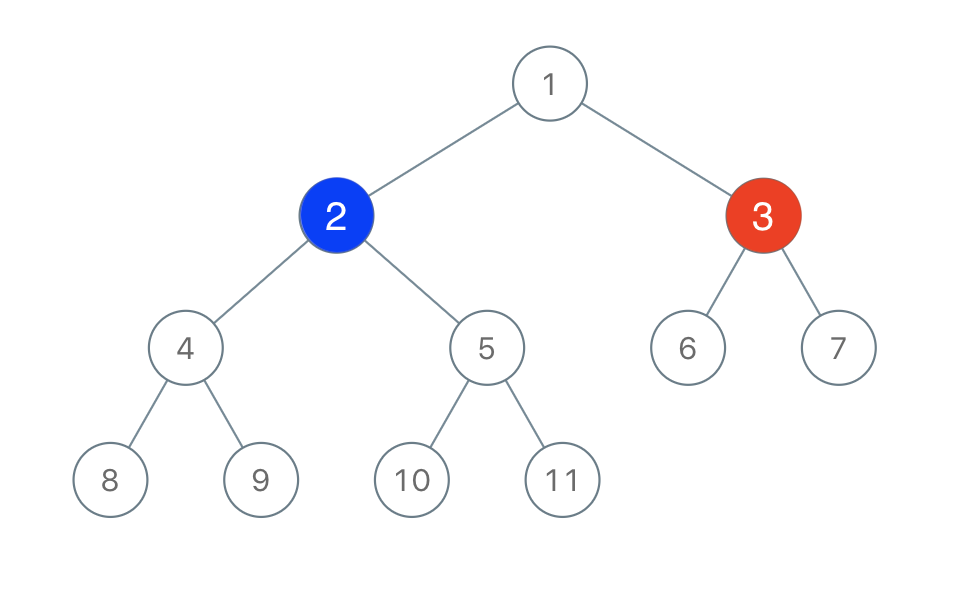# 二叉树着色游戏

## 1145. 二叉树着色游戏 (Medium)

「一号」玩家从 `[1, n]` 中取一个值 `x``1 <= x <= n`）；

「二号」玩家也从 `[1, n]` 中取一个值 `y``1 <= y <= n`）且 `y != x`

「一号」玩家给值为 `x` 的节点染上红色，而「二号」玩家给值为 `y` 的节点染上蓝色。```输入：root = [1,2,3,4,5,6,7,8,9,10,11], n = 11, x = 3

```

• 二叉树的根节点为 `root`，树上由 `n` 个节点，节点上的值从 `1``n` 各不相同。
• `n` 为奇数。
• `1 <= x <= n <= 100`

### 相关话题

[] [深度优先搜索] [二叉树]